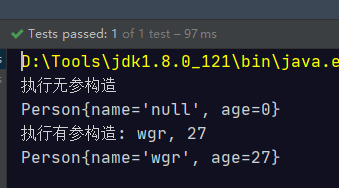# Jdk8新特性之方法引用

1. instanceName::methodName 对象::方法名
2. ClassName::staticMethodName 类名::静态方法
3. ClassName::methodName 类名::普通方法
4. ClassName::new 类名::new 调用的构造器
5. TypeName[]::new String[]::new 调用数组的构造器

// 对象::实例方法
@Test
public void test01() {
Date d = new Date();
Supplier<Long> getTime = d::getTime;
Long time = getTime.get();
System.out.println(time);
}

1. 被引用的方法，参数要和接口中抽象方法的参数一样
2. 当接口抽象方法有返回值时，被引用的方法也必须有返回值

    // 类名::静态方法
@Test
public void test02() {
//1585110320763
Supplier<Long> timeMillis = System::currentTimeMillis;
System.out.println(timeMillis.get());
}

Java面向对象中，类名只能调用静态方法，类名引用实例方法是有前提的，实际上是拿第一个参数作为方法的调用者。

    // 类名::实例方法
@Test
public void test03() {
//1
Function<String, Integer> length = String::length;
System.out.println(length.apply("hello"));   // 5

Function<String, Integer> length1 = s1 -> {
return s1.length();
};

//2
BiFunction<String, Integer, String> substring = String::substring;
String s = substring.apply("hello", 3);  //lo
System.out.println(s);

BiFunction<String, Integer, String> substr = (String s1, Integer i1) -> {
return s1.substring(i1);
};
String hello = substr.apply("hello", 3);
System.out.println(hello);
}

// 类名::new引用类的构造器
@Test
public void test04() {
Supplier<Person> p1 = Person::new;
Person person = p1.get();
System.out.println(person);
BiFunction<String, Integer, Person> p2 = Person::new;
Person wgr = p2.apply("wgr", 27);
System.out.println(wgr);
}// 类型[]::new
@Test
public void test05() {
Function<Integer, int[]> f1 = int[]::new;

int[] arr1 = f1.apply(10);
System.out.println(Arrays.toString(arr1));
}

©️2019 CSDN 皮肤主题: 大白 设计师: CSDN官方博客